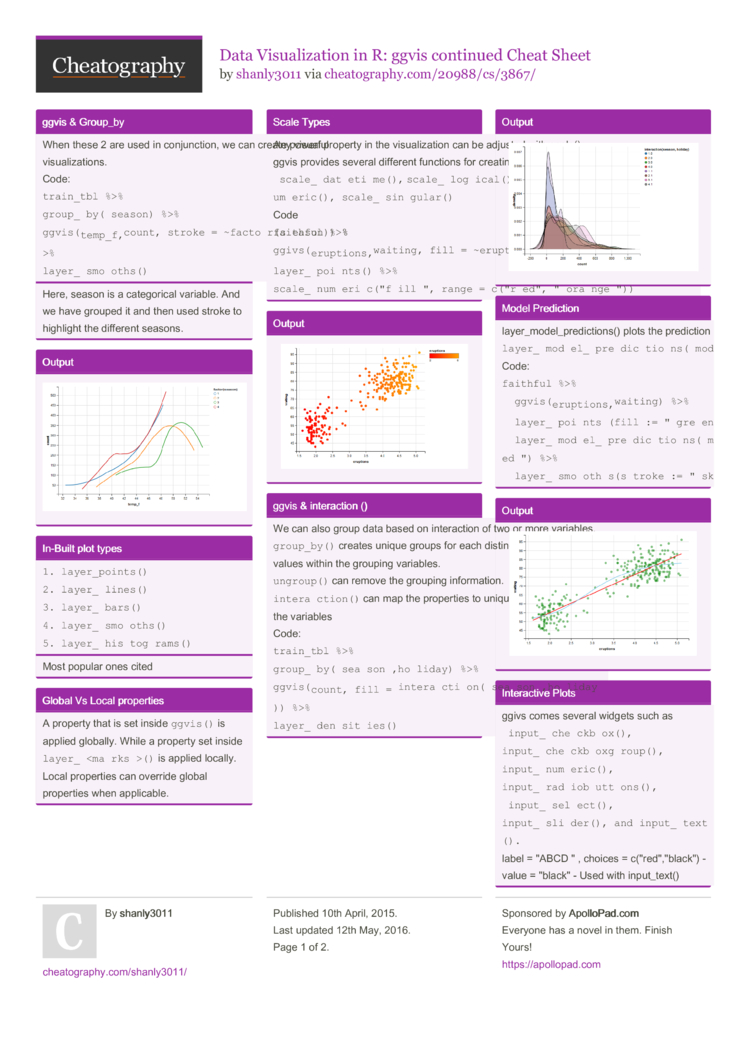# Data Visualization in R: ggvis continued Cheat Sheet by shanly3011

### ggvis & Group_by

 When these 2 are used in conjun­ction, we can create powerful visual­iza­tions. Code: `train_tbl %>%` `group­_by­(se­ason) %>%` `ggvis­(~t­emp­_f,­~count, stroke = ~facto­r(s­eason)) %>%` `layer­_sm­oot­hs()`
Here, season is a catego­rical variable. And we have grouped it and then used stroke to highlight the different seasons.

### Output### In-Built plot types

 ```1. layer_points() 2. layer_lines() 3. layer_bars() 4. layer_smooths() 5. layer_histograms()```
Most popular ones cited

### Global Vs Local properties

 A property that is set inside `ggvis()` is applied globally. While a property set inside `layer­_()` is applied locally. Local properties can override global properties when applic­able.

### Scale Types

 Any visual property in the visual­ization can be adjusted with scale(). ggvis provides several different functions for creating scales: ` scale_­dat­eti­me(),` `scale­_lo­gic­al(), scale_­nom­inal(), scale_­num­eric(), scale_­sin­gul­ar()` Code `faithful %>%` `ggivs­(~e­rup­tio­ns,­~wa­iting, fill = ~erupt­ions) %>%` `layer­_po­ints() %>%` `scale­_nu­mer­ic(­"­fil­l", range = c("r­ed",­"­ora­nge­"))`

### Output### ggvis & intera­ction ()

 We can also group data based on intera­ction of two or more variables. `group­_by()` creates unique groups for each distinct combin­ation of values within the grouping variables. `ungro­up()` can remove the grouping inform­ation. `inter­act­ion()` can map the properties to unique combin­ations of the variables Code: `train_tbl %>%` `group­_by­(se­aso­n,h­oliday) %>%` `ggvis­(~c­ount, fill = ~inter­act­ion­(se­aso­n,h­oli­day)) %>%` `layer­_de­nsi­ties()`

### Output### Model Prediction

 layer_­mod­el_­pre­dic­tions() plots the prediction line of a model fitted to the data. `layer­_mo­del­_pr­edi­cti­ons­(model = "­lm")` Code: `faithful %>%` ` ggvis(­~er­upt­ion­s,~­wai­ting) %>%` ` layer_­poi­nts­(fill := "­gre­en", fillOp­acity := 0.5) %>%` ` layer_­mod­el_­pre­dic­tio­ns(­model = "­lm", stroke := "­red­") %>%` ` layer_­smo­oth­s(s­troke := "­sky­blu­e")`

### Intera­ctive Plots

 ggivs comes several widgets such as ` input_­che­ckb­ox(), ` `input­_ch­eck­box­gro­up(), ` `input­_nu­mer­ic(), ` `input­_ra­dio­but­ton­s(),` ` input_­sel­ect(), ` `input­_sl­ider(), and input_­tex­t().` label = "ABCD " , choices = c("r­ed",­"­bla­ck") - value = "­bla­ck" - Used with input_­text() map = as.name used when we want to return variable names Are the common arguments inside these functions.

### Output### Legends & Axis

 Axis You can add axes with `add_a­xis()` Syntax: `faithful %>%` `ggvis­(~e­rup­tio­ns,­~wa­iting) %>%` `add_a­xis­("x", label = "­Eru­pti­ons­", values = c(1,2,­3,4), subdivide = 9, orient = ``top") %>%` `layer­_po­ints()` Lege­nds `ggvis` adds a legend for each property that is specified. To combine multiple legends into a single legend with common values, use a vector of property names. `add_l­ege­nd()` `hide_­leg­end()` Syntax `faithful %>% ` ` ggvis(­~wa­iting, ~erupt­ions, opacity := 0.6, ` ` fill = ~facto­r(r­oun­d(e­rup­tio­ns)), shape = ~facto­r(r­oun­d(e­rup­tio­ns)), ` ` size = ~round­(er­upt­ions)) %>% ` ` layer_­poi­nts() %>% ` ` add_le­gen­d(c­("fi­ll", "­sha­pe", "­siz­e"), ` ` title = "~ duration (m)", values = c(2, 3, 4, 5))`2 Pages
//media.cheatography.com/storage/thumb/shanly3011_data-visualization-in-r-ggvis-continued.750.jpg

PDF (recommended)